# Ginzburg-Landau equation

(diff) ← Older revision | Latest revision (diff) | Newer revision → (diff)

A modulation (or amplitude/envelope equation) that describes the evolution of small perturbations of a marginally unstable basic state of a system of non-linear partial differential equations on an unbounded domain (cf. also Perturbation theory; Perturbation of a linear system; Linear partial differential equation). The stationary problem associated to the Ginzburg–Landau equation with real coefficients also has a different background as the Euler–Lagrange equation associated to the Ginzburg–Landau functional (see below). To obtain the Ginzburg–Landau equation as modulation equation, one lets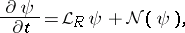(a1)describe an underlying problem (with certain boundary conditions), where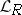is an elliptic linear operator,a non-linear operator of order less than,a (bifurcation) parameter anda bounded domain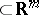. The linearized stability of the basic solution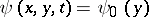of (a1) is determined by setting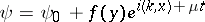and solving an eigenvalue problem foronfor any pair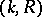(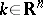). Under certain conditions on the eigenvalue problem, one can define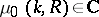as the critical eigenvalue (i.e.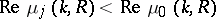for all,and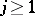) and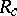as the critical value of(for alland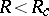, i.e. the neutral manifold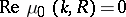has a minimum at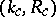in-space:is linearly stable for). Introduce,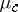byandas the critical eigenfunction at,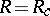. For,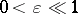,can be expanded both as an asymptotic series and as a Fourier series: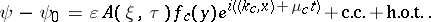Here,is an unknown amplitude andandare rescaled variables: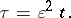The Ginzburg–Landau equation describes the evolution of:(a2)(at leading order). The equation is obtained (formally) by inserting the above expansion into (a1) and applying an orthogonality condition. The second-order differential operator is elliptic whenis an isolated non-degenerate minimum of the neutral manifold. The Landau constant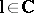can be expressed in terms of information obtained from the linear eigenvalue problem and its adjoint. In most cases studied in the literature,and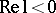; (a2) can then be rescaled into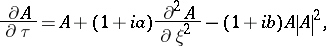(a3)

with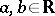. Historically, the Ginzburg–Landau equation was first derived as a modulation equation for two classical hydrodynamic stability problems: Rayleigh–Bénard convection [a4] and Poiseuille flow [a5]. Several aspects of the mathematical validity of this formal approximation scheme have been studied in [a2], [a7], [a6].

By its nature, the Ginzburg–Landau equation appears as leading-order approximation in many systems. Therefore, there is much literature on the behaviour of its solutions. Its most simple solutions are periodic: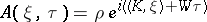. Under certain conditions on the coefficients there is a subfamily of stable periodic solutions; it is called the Eckhaus band in (a3). The existence and stability of more complicated "localized" (homoclinic, heteroclinic) solutions to (a3) (also with more general non-linear terms) is considered in [a8] (with mostly formal results). Up to now (1998), there is no mathematical text (book or survey paper) that gives an overview of what is known about the behaviour of solutions of the Ginzburg–Landau equation as evolution equation onor even on.

However, much is known about the solutions of the stationary (elliptic) Ginzburg–Landau equation with real coefficients on bounded domains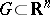[a1]. The Ginzburg–Landau equation with real coefficients has a variational structure: the Ginzburg–Landau functional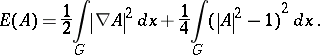The Ginzburg–Landau functional appears in various parts of science; in general, it is not related to the above sketched modulation equation interpretation of the Ginzburg–Landau equation [a1]. The name "Ginzburg–Landau" , both of the equation and of the functional, comes from a paper on superconductivity [a3]. However, in this context the (real, stationary) equation and/or the functional is part of a larger system of equations/functionals.

How to Cite This Entry:
Ginzburg-Landau equation. Encyclopedia of Mathematics. URL: http://encyclopediaofmath.org/index.php?title=Ginzburg-Landau_equation&oldid=16717
This article was adapted from an original article by Arjen Doelman (originator), which appeared in Encyclopedia of Mathematics - ISBN 1402006098. See original article